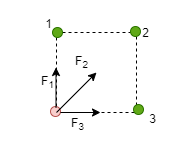# Problem: Three identical charges q are placed at each of three corners of a square of side L. Find the magnitude of the net force on a point charge -3q placed at the vacant corner of the square. Draw a free-body diagram showing the forces exerted on the -3q charge by each of the other three charges.

###### FREE Expert Solution

Electric force:

$\overline{){\mathbf{F}}{\mathbf{=}}\frac{\mathbf{k}{\mathbf{q}}_{\mathbf{1}}{\mathbf{q}}_{\mathbf{2}}}{{\mathbf{r}}^{\mathbf{2}}}}$

Consider the free-body diagram:The force due to -3q located at the vacant point is obtained as follows:

Force due to charge at point 1

F1 = kq(3q)/L2 = 3kq2/L2

82% (414 ratings)###### Problem Details

Three identical charges q are placed at each of three corners of a square of side L. Find the magnitude of the net force on a point charge -3q placed at the vacant corner of the square. Draw a free-body diagram showing the forces exerted on the -3q charge by each of the other three charges.